# Mean State

Period Mean (original grids) [W/m2]
Model Period Mean (intersection) [W/m2]
Model Period Mean (complement) [W/m2]
Benchmark Period Mean (intersection) [W/m2]
Benchmark Period Mean (complement) [W/m2]
Bias [W/m2]
RMSE [W/m2]
Phase Shift [months]
Bias Score 
RMSE Score 
Seasonal Cycle Score 
Spatial Distribution Score 
Interannual Variability Score 
Overall Score 
Benchmark [-] 401.
CRUNCEPv7 [-] 408. 408. 0.00 400. 415. 9.76 15.2 1.36 0.29 0.34 0.82 0.94 0.74 0.58
GSWP3v1 [-] 397. 398. 0.00 400. 415. -1.37 9.58 1.81 0.50 0.39 0.73 0.91 0.65 0.60
WFDEI [-] 417. 418. 0.00 400. 415. 19.0 25.9 1.23 0.16 0.20 0.83 0.81 0.47 0.45
Period Mean (original grids) [W/m2]
Model Period Mean (intersection) [W/m2]
Model Period Mean (complement) [W/m2]
Benchmark Period Mean (intersection) [W/m2]
Benchmark Period Mean (complement) [W/m2]
Bias [W/m2]
RMSE [W/m2]
Phase Shift [months]
Bias Score 
RMSE Score 
Seasonal Cycle Score 
Spatial Distribution Score 
Interannual Variability Score 
Overall Score 
Benchmark [-] 391.
CRUNCEPv7 [-] 397. 397. 0.00 391. 404. 6.63 15.0 0.817 0.67 0.65 0.91 0.97 0.77 0.77
GSWP3v1 [-] 386. 386. 0.00 391. 404. -4.87 12.4 0.491 0.73 0.66 0.95 0.91 0.71 0.77
WFDEI [-] 402. 401. 0.00 391. 404. 10.6 24.8 0.981 0.59 0.45 0.88 0.93 0.60 0.65
Period Mean (original grids) [W/m2]
Model Period Mean (intersection) [W/m2]
Model Period Mean (complement) [W/m2]
Benchmark Period Mean (intersection) [W/m2]
Benchmark Period Mean (complement) [W/m2]
Bias [W/m2]
RMSE [W/m2]
Phase Shift [months]
Bias Score 
RMSE Score 
Seasonal Cycle Score 
Spatial Distribution Score 
Interannual Variability Score 
Overall Score 
Benchmark [-] 367.
CRUNCEPv7 [-] 370. 370. 0.00 366. 384. 6.16 15.0 1.19 0.61 0.62 0.85 0.99 0.73 0.74
GSWP3v1 [-] 361. 361. 0.00 366. 384. -2.96 12.2 0.935 0.71 0.62 0.88 0.99 0.75 0.76
WFDEI [-] 368. 368. 0.00 366. 384. 3.88 18.3 1.18 0.63 0.51 0.86 0.97 0.77 0.71
Period Mean (original grids) [W/m2]
Model Period Mean (intersection) [W/m2]
Model Period Mean (complement) [W/m2]
Benchmark Period Mean (intersection) [W/m2]
Benchmark Period Mean (complement) [W/m2]
Bias [W/m2]
RMSE [W/m2]
Phase Shift [months]
Bias Score 
RMSE Score 
Seasonal Cycle Score 
Spatial Distribution Score 
Interannual Variability Score 
Overall Score 
Benchmark [-] 243.
CRUNCEPv7 [-] 207. 207. 0.00 242. 256. -32.7 34.1 0.143 0.57 0.82 0.99 0.99 0.72 0.82
GSWP3v1 [-] 237. 237. 0.00 242. 256. -2.60 13.7 0.123 0.92 0.80 0.99 0.99 0.89 0.90
WFDEI [-] 206. 206. 0.00 242. 256. -34.1 37.4 0.125 0.56 0.75 0.99 1.0 0.78 0.81
Period Mean (original grids) [W/m2]
Model Period Mean (intersection) [W/m2]
Model Period Mean (complement) [W/m2]
Benchmark Period Mean (intersection) [W/m2]
Benchmark Period Mean (complement) [W/m2]
Bias [W/m2]
RMSE [W/m2]
Phase Shift [months]
Bias Score 
RMSE Score 
Seasonal Cycle Score 
Spatial Distribution Score 
Interannual Variability Score 
Overall Score 
Benchmark [-] 411.
CRUNCEPv7 [-] 427. 427. 0.00 409. 416. 20.6 23.6 2.39 0.084 0.35 0.62 0.98 0.66 0.51
GSWP3v1 [-] 411. 411. 0.00 409. 416. 3.75 11.6 1.88 0.37 0.37 0.71 0.57 0.75 0.52
WFDEI [-] 438. 438. 0.00 409. 416. 29.5 31.3 2.50 0.054 0.24 0.61 0.99 0.56 0.45
Period Mean (original grids) [W/m2]
Model Period Mean (intersection) [W/m2]
Model Period Mean (complement) [W/m2]
Benchmark Period Mean (intersection) [W/m2]
Benchmark Period Mean (complement) [W/m2]
Bias [W/m2]
RMSE [W/m2]
Phase Shift [months]
Bias Score 
RMSE Score 
Seasonal Cycle Score 
Spatial Distribution Score 
Interannual Variability Score 
Overall Score 
Benchmark [-] 268.
CRUNCEPv7 [-] 245. 245. 0.00 267. 280. -22.2 23.6 0.0562 0.68 0.84 1.0 1.0 0.88 0.87
GSWP3v1 [-] 265. 264. 0.00 267. 280. -2.61 10.9 0.0463 0.92 0.85 1.0 0.97 0.86 0.91
WFDEI [-] 243. 243. 0.00 267. 280. -24.3 28.9 0.0443 0.66 0.75 1.0 1.0 0.87 0.84
Period Mean (original grids) [W/m2]
Model Period Mean (intersection) [W/m2]
Model Period Mean (complement) [W/m2]
Benchmark Period Mean (intersection) [W/m2]
Benchmark Period Mean (complement) [W/m2]
Bias [W/m2]
RMSE [W/m2]
Phase Shift [months]
Bias Score 
RMSE Score 
Seasonal Cycle Score 
Spatial Distribution Score 
Interannual Variability Score 
Overall Score 
Benchmark [-] 297.
CRUNCEPv7 [-] 280. 279. 0.00 296. 341. -13.5 20.1 0.119 0.74 0.78 0.99 1.0 0.82 0.85
GSWP3v1 [-] 293. 292. 0.00 296. 341. -0.587 14.2 0.144 0.84 0.81 0.99 0.99 0.79 0.87
WFDEI [-] 280. 280. 0.00 296. 341. -13.2 23.1 0.135 0.73 0.71 0.99 1.0 0.84 0.83
Period Mean (original grids) [W/m2]
Model Period Mean (intersection) [W/m2]
Model Period Mean (complement) [W/m2]
Benchmark Period Mean (intersection) [W/m2]
Benchmark Period Mean (complement) [W/m2]
Bias [W/m2]
RMSE [W/m2]
Phase Shift [months]
Bias Score 
RMSE Score 
Seasonal Cycle Score 
Spatial Distribution Score 
Interannual Variability Score 
Overall Score 
Benchmark [-] 387.
CRUNCEPv7 [-] 386. 387. 0.00 385. 415. 8.18 20.7 0.893 0.59 0.68 0.91 0.96 0.72 0.76
GSWP3v1 [-] 374. 375. 0.00 385. 415. -5.63 15.4 0.408 0.72 0.71 0.96 1.0 0.80 0.82
WFDEI [-] 390. 391. 0.00 385. 415. 11.8 24.5 0.839 0.56 0.58 0.92 0.97 0.75 0.73
Period Mean (original grids) [W/m2]
Model Period Mean (intersection) [W/m2]
Model Period Mean (complement) [W/m2]
Benchmark Period Mean (intersection) [W/m2]
Benchmark Period Mean (complement) [W/m2]
Bias [W/m2]
RMSE [W/m2]
Phase Shift [months]
Bias Score 
RMSE Score 
Seasonal Cycle Score 
Spatial Distribution Score 
Interannual Variability Score 
Overall Score 
Benchmark [-] 249.
CRUNCEPv7 [-] 217. 217. 0.00 248. 253. -31.0 31.9 0.0590 0.57 0.83 1.0 1.0 0.79 0.84
GSWP3v1 [-] 243. 244. 0.00 248. 253. -4.14 13.6 0.0505 0.92 0.80 1.0 0.98 0.91 0.90
WFDEI [-] 214. 215. 0.00 248. 253. -33.5 37.5 0.0532 0.55 0.72 1.0 1.0 0.85 0.81
Period Mean (original grids) [W/m2]
Model Period Mean (intersection) [W/m2]
Model Period Mean (complement) [W/m2]
Benchmark Period Mean (intersection) [W/m2]
Benchmark Period Mean (complement) [W/m2]
Bias [W/m2]
RMSE [W/m2]
Phase Shift [months]
Bias Score 
RMSE Score 
Seasonal Cycle Score 
Spatial Distribution Score 
Interannual Variability Score 
Overall Score 
Benchmark [-] 353.
CRUNCEPv7 [-] 352. 352. 0.00 352. 359. 0.323 13.5 0.455 0.79 0.71 0.96 0.95 0.76 0.81
GSWP3v1 [-] 351. 351. 0.00 352. 359. -0.968 9.75 0.299 0.86 0.76 0.98 0.99 0.87 0.87
WFDEI [-] 352. 352. 0.00 352. 359. -0.00944 17.5 0.620 0.80 0.61 0.95 0.95 0.86 0.80
Period Mean (original grids) [W/m2]
Model Period Mean (intersection) [W/m2]
Model Period Mean (complement) [W/m2]
Benchmark Period Mean (intersection) [W/m2]
Benchmark Period Mean (complement) [W/m2]
Bias [W/m2]
RMSE [W/m2]
Phase Shift [months]
Bias Score 
RMSE Score 
Seasonal Cycle Score 
Spatial Distribution Score 
Interannual Variability Score 
Overall Score 
Benchmark [-] 314.
CRUNCEPv7 [-] 292. 292. 0.00 312. 329. -16.4 21.0 0.237 0.58 0.71 0.98 0.87 0.84 0.79
GSWP3v1 [-] 306. 306. 0.00 312. 329. -3.25 11.6 0.223 0.81 0.75 0.98 1.0 0.70 0.83
WFDEI [-] 289. 289. 0.00 312. 329. -20.2 26.5 0.385 0.53 0.61 0.97 0.87 0.87 0.74
Period Mean (original grids) [W/m2]
Model Period Mean (intersection) [W/m2]
Model Period Mean (complement) [W/m2]
Benchmark Period Mean (intersection) [W/m2]
Benchmark Period Mean (complement) [W/m2]
Bias [W/m2]
RMSE [W/m2]
Phase Shift [months]
Bias Score 
RMSE Score 
Seasonal Cycle Score 
Spatial Distribution Score 
Interannual Variability Score 
Overall Score 
Benchmark [-] 345.
CRUNCEPv7 [-] 307. 307. 0.00 315. 360. -5.27 20.9 0.667 0.59 0.65 0.92 0.99 0.73 0.75
GSWP3v1 [-] 310. 310. 0.00 315. 360. -2.77 13.1 0.509 0.76 0.69 0.94 1.0 0.80 0.81
WFDEI [-] 306. 306. 0.00 315. 360. -6.07 24.7 0.718 0.58 0.56 0.91 0.99 0.77 0.73
Period Mean (original grids) [W/m2]
Model Period Mean (intersection) [W/m2]
Model Period Mean (complement) [W/m2]
Benchmark Period Mean (intersection) [W/m2]
Benchmark Period Mean (complement) [W/m2]
Bias [W/m2]
RMSE [W/m2]
Phase Shift [months]
Bias Score 
RMSE Score 
Seasonal Cycle Score 
Spatial Distribution Score 
Interannual Variability Score 
Overall Score 
Benchmark [-] 350.
CRUNCEPv7 [-] 353. 353. 0.00 349. 373. 6.08 16.6 0.412 0.76 0.74 0.97 0.97 0.71 0.81
GSWP3v1 [-] 344. 344. 0.00 349. 373. -3.78 14.4 0.431 0.82 0.73 0.96 0.99 0.79 0.84
WFDEI [-] 340. 340. 0.00 349. 373. -7.45 19.9 0.392 0.76 0.65 0.97 1.0 0.78 0.80
Period Mean (original grids) [W/m2]
Model Period Mean (intersection) [W/m2]
Model Period Mean (complement) [W/m2]
Benchmark Period Mean (intersection) [W/m2]
Benchmark Period Mean (complement) [W/m2]
Bias [W/m2]
RMSE [W/m2]
Phase Shift [months]
Bias Score 
RMSE Score 
Seasonal Cycle Score 
Spatial Distribution Score 
Interannual Variability Score 
Overall Score 
Benchmark [-] 248.
CRUNCEPv7 [-] 218. 218. 0.00 247. 258. -29.9 31.1 0.153 0.57 0.81 0.99 0.99 0.69 0.81
GSWP3v1 [-] 243. 243. 0.00 247. 258. -4.19 13.4 0.126 0.90 0.79 0.99 1.0 0.90 0.90
WFDEI [-] 216. 216. 0.00 247. 258. -31.9 35.6 0.145 0.55 0.72 0.99 0.98 0.82 0.80
Period Mean (original grids) [W/m2]
Model Period Mean (intersection) [W/m2]
Model Period Mean (complement) [W/m2]
Benchmark Period Mean (intersection) [W/m2]
Benchmark Period Mean (complement) [W/m2]
Bias [W/m2]
RMSE [W/m2]
Phase Shift [months]
Bias Score 
RMSE Score 
Seasonal Cycle Score 
Spatial Distribution Score 
Interannual Variability Score 
Overall Score 
Benchmark [-] 376.
CRUNCEPv7 [-] 373. 373. 0.00 371. 402. 12.1 20.3 0.938 0.49 0.63 0.89 0.97 0.74 0.73
GSWP3v1 [-] 363. 363. 0.00 371. 402. -1.23 14.5 0.704 0.64 0.68 0.91 0.99 0.78 0.78
WFDEI [-] 376. 376. 0.00 371. 402. 13.3 23.0 0.751 0.49 0.54 0.93 0.98 0.79 0.71
Period Mean (original grids) [W/m2]
Model Period Mean (intersection) [W/m2]
Model Period Mean (complement) [W/m2]
Benchmark Period Mean (intersection) [W/m2]
Benchmark Period Mean (complement) [W/m2]
Bias [W/m2]
RMSE [W/m2]
Phase Shift [months]
Bias Score 
RMSE Score 
Seasonal Cycle Score 
Spatial Distribution Score 
Interannual Variability Score 
Overall Score 
Benchmark [-] 335.
CRUNCEPv7 [-] 322. 322. 0.00 333. 372. -7.59 16.6 0.145 0.79 0.77 0.99 0.92 0.89 0.86
GSWP3v1 [-] 328. 328. 0.00 333. 372. -2.37 9.63 0.122 0.92 0.83 0.99 1.0 0.81 0.90
WFDEI [-] 318. 318. 0.00 333. 372. -11.8 22.5 0.169 0.77 0.68 0.99 0.93 0.82 0.81
Period Mean (original grids) [W/m2]
Model Period Mean (intersection) [W/m2]
Model Period Mean (complement) [W/m2]
Benchmark Period Mean (intersection) [W/m2]
Benchmark Period Mean (complement) [W/m2]
Bias [W/m2]
RMSE [W/m2]
Phase Shift [months]
Bias Score 
RMSE Score 
Seasonal Cycle Score 
Spatial Distribution Score 
Interannual Variability Score 
Overall Score 
Benchmark [-] 278.
CRUNCEPv7 [-] 255. 255. 0.00 277. 303. -21.0 22.3 0.0800 0.62 0.81 0.99 1.0 0.87 0.85
GSWP3v1 [-] 275. 275. 0.00 277. 303. -0.971 9.62 0.0673 0.91 0.82 1.0 0.99 0.86 0.90
WFDEI [-] 253. 253. 0.00 277. 303. -22.8 27.7 0.104 0.60 0.68 0.99 1.0 0.84 0.80
Period Mean (original grids) [W/m2]
Model Period Mean (intersection) [W/m2]
Model Period Mean (complement) [W/m2]
Benchmark Period Mean (intersection) [W/m2]
Benchmark Period Mean (complement) [W/m2]
Bias [W/m2]
RMSE [W/m2]
Phase Shift [months]
Bias Score 
RMSE Score 
Seasonal Cycle Score 
Spatial Distribution Score 
Interannual Variability Score 
Overall Score 
Benchmark [-] 298.
CRUNCEPv7 [-] 287. 289. 0.00 298. 325. -8.55 16.3 0.107 0.72 0.76 0.99 0.96 0.80 0.83
GSWP3v1 [-] 293. 294. 0.00 298. 325. -3.00 12.5 0.124 0.83 0.76 0.99 0.99 0.74 0.85
WFDEI [-] 287. 288. 0.00 298. 325. -9.15 18.8 0.335 0.74 0.67 0.98 0.99 0.80 0.81
Period Mean (original grids) [W/m2]
Model Period Mean (intersection) [W/m2]
Model Period Mean (complement) [W/m2]
Benchmark Period Mean (intersection) [W/m2]
Benchmark Period Mean (complement) [W/m2]
Bias [W/m2]
RMSE [W/m2]
Phase Shift [months]
Bias Score 
RMSE Score 
Seasonal Cycle Score 
Spatial Distribution Score 
Interannual Variability Score 
Overall Score 
Benchmark [-] 410.
CRUNCEPv7 [-] 426. 426. 0.00 410. 415. 16.9 23.1 1.99 0.18 0.38 0.71 0.82 0.82 0.55
GSWP3v1 [-] 406. 406. 0.00 410. 415. -2.98 10.8 1.26 0.55 0.47 0.84 0.98 0.77 0.68
WFDEI [-] 432. 432. 0.00 410. 415. 23.2 28.5 2.49 0.13 0.27 0.61 0.85 0.60 0.45
Period Mean (original grids) [W/m2]
Model Period Mean (intersection) [W/m2]
Model Period Mean (complement) [W/m2]
Benchmark Period Mean (intersection) [W/m2]
Benchmark Period Mean (complement) [W/m2]
Bias [W/m2]
RMSE [W/m2]
Phase Shift [months]
Bias Score 
RMSE Score 
Seasonal Cycle Score 
Spatial Distribution Score 
Interannual Variability Score 
Overall Score 
Benchmark [-] 379.
CRUNCEPv7 [-] 386. 385. 0.00 380. 374. 6.23 15.3 1.60 0.61 0.62 0.76 0.95 0.78 0.72
GSWP3v1 [-] 377. 376. 0.00 380. 374. -2.98 11.2 0.680 0.77 0.65 0.94 0.99 0.84 0.81
WFDEI [-] 385. 385. 0.00 380. 374. 5.55 18.3 1.50 0.63 0.49 0.79 0.98 0.82 0.70
Period Mean (original grids) [W/m2]
Model Period Mean (intersection) [W/m2]
Model Period Mean (complement) [W/m2]
Benchmark Period Mean (intersection) [W/m2]
Benchmark Period Mean (complement) [W/m2]
Bias [W/m2]
RMSE [W/m2]
Phase Shift [months]
Bias Score 
RMSE Score 
Seasonal Cycle Score 
Spatial Distribution Score 
Interannual Variability Score 
Overall Score 
Benchmark [-] 303.
CRUNCEPv7 [-] 289. 288. 0.00 298. 346. -5.88 22.2 0.555 0.49 0.54 0.95 0.98 0.68 0.69
GSWP3v1 [-] 301. 301. 0.00 298. 346. 6.58 22.1 0.556 0.48 0.53 0.95 0.93 0.75 0.70
WFDEI [-] 298. 297. 0.00 298. 346. 1.85 24.2 0.632 0.47 0.47 0.95 0.98 0.75 0.68

# Temporally integrated period mean

BENCHMARK MEAN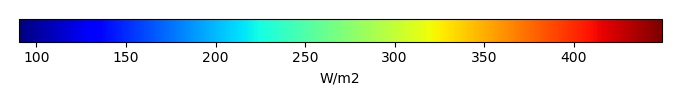MODEL MEANBIAS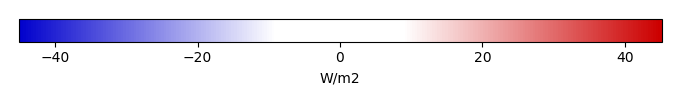BIAS SCORERMSE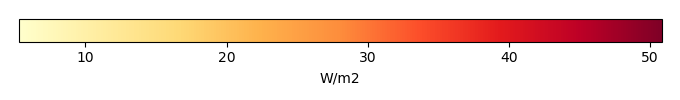RMSE SCOREBENCHMARK INTERANNUAL VARIABILITY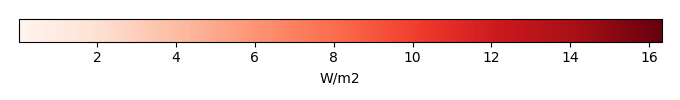MODEL INTERANNUAL VARIABILITYINTERANNUAL VARIABILITY SCOREBENCHMARK MAX MONTHMODEL MAX MONTHDIFFERENCE IN MAX MONTH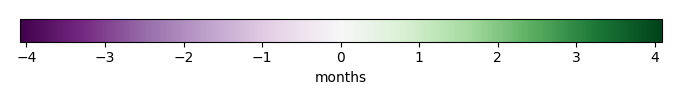SEASONAL CYCLE SCORESPATIAL TAYLOR DIAGRAMMODEL COLORS# Spatially integrated regional mean

MODEL COLORSREGIONAL MEANANNUAL CYCLEMONTHLY ANOMALYANNUAL CYCLE# All Models

BenchmarkCRUNCEPv7GSWP3v1WFDEI# Data Information

creation_date: Thu May 8 23:18:00 PDT 2014

source_file: This product is generated from monthly 1 degree CERES EBAF Radiation observations